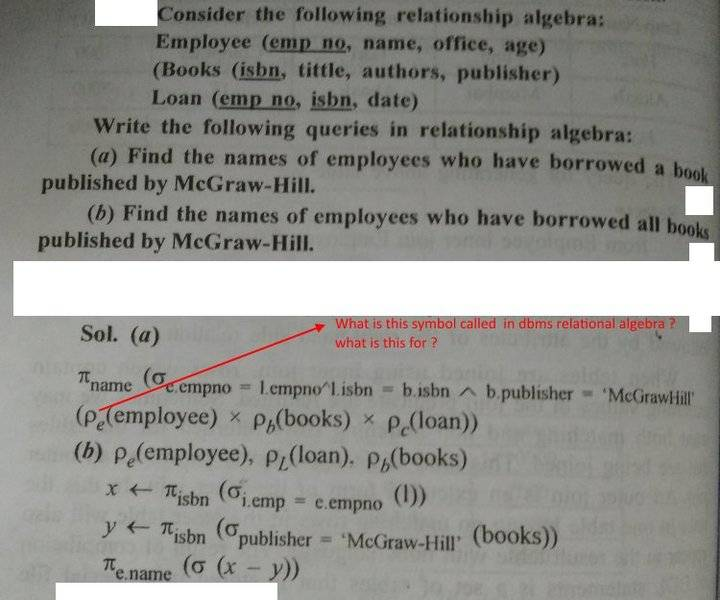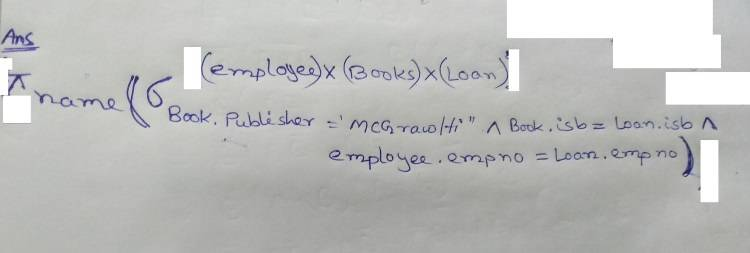# Cartesian product

<Moderator's note: Moved from a technical forum and thus no template.>

Here is a problem and solution given in my book.

this is using a symbol in cartesian product .

I checked other books for cartesian product examples but there was no such symbol being used.Here is my solution without that symbolIs my solution wrong ?

I'm confused.

#### Attachments

Last edited by a moderator:

verty
Homework Helper
I just looked this up. That is the greek character rho (##\rho##) which means rename according to wikipedia. So employee is renamed to e, books to b, loan to c, then it creates the cartesian product. Does that help?

I just looked this up. That is the greek character rho (##\rho##) which means rename according to wikipedia. So employee is renamed to e, books to b, loan to c, then it creates the cartesian product. Does that help?
okay. But is my solution correct ?

I am not renaming. Can I do this ?

verty
Homework Helper
I don't want to mislead you but for some reason I have an inkling that it may be necessary in the relational algebra to use rename when attributes would clash. So for example, if you were joining from employee to employee and matching each staff member to their manager, it would be necessary to use rename. I think in this problem it isn't necessary.

Why did you write employee x books x loan at the top? Should it not be in line with the rest?

fresh_42
Mentor
2021 Award
<Moderator's note: Moved from a technical forum and thus no template.>

Here is a problem and solution given in my book.

this is using a symbol in cartesian product .

I checked other books for cartesian product examples but there was no such symbol being used.

View attachment 232159

Here is my solution without that symbol
View attachment 232160

Is my solution wrong ?

I'm confused.

Without more context, especially the definition of a relation algebra, it is hard to tell.
##\rho## is the Greek letter ##r##, so one might suspect that it stands for relation. Also is it indexed by the three categories, which might indicate that ##\rho_{\text{employee}} \times \rho_{\text{books}} \times \rho_{\text{loan}}## is simply the phase space of all possible relations, resp. their tables in the database.

•momentum
Why did you write employee x books x loan at the top? Should it not be in line with the rest?
I believe notation of sigma says, at the top you put name of the relation and at bottom you put the condition. There is no in line stuff with this syntax. Is it wrong ?

verty
Homework Helper
I believe notation of sigma says, at the top you put name of the relation and at bottom you put the condition. There is no in line stuff with this syntax. Is it wrong ?

Wow I'm confused by your how your book has written these answers. I believe the answer to A given in the book should look like this (there was a typo):
$$\pi_{name} (\sigma_{e.empno = l.empno \land l.isbn = b.isbn \land b.publisher = 'McGraw-Hill'} (\rho_e(employee) \times \rho_l(loan) \times \rho_b(books)))$$
And I don't know what is going on with answer B. It just doesn't look right to me.

In your answer, I don't think putting the relation at the top is correct. Sorry, I'm doing the best I can to help.

Wow I'm confused by your how your book has written these answers. I believe the answer to A given in the book should look like this:
$$\pi_{name} (\sigma_{e.empno = l.empno \land l.isbn = b.isbn \land b.publisher = 'McGraw Hill'} (\rho_e(employee) \times \rho_l(loan) \times \rho_b(books)))$$
And I don't know what is going on with answer B. It just doesn't look right to me.

In your answer, I don't think putting the relation at the top is correct. Sorry, I'm doing the best I can to help.

okay . I understand answer to A now.

But I am confused with answer to B.

What does these mean ?
x<--
y<---

I checked relational algebra notations but could not find such notations. And so I'm stuck to understand solution B .
If solution B is not correct solution. What is the right solution ?

fresh_42
Mentor
2021 Award
okay . I understand answer to A now.

But I am confused with answer to B.

What does these mean ?
x<--
y<---

I checked relational algebra notations but could not find such notations. And so I'm stuck to understand solution B .
If this is not correct solution. What is the right solution ?
I thought it meant "Select ... as x , select ... as y , ... where ... " but I don't understand the minus in ##x-y##. Shouldn't it be an ##AND\,?##

verty
Homework Helper
B should be something like:
##\rho_e(employee), \rho_l(loan), \rho_b(books)##
##x \leftarrow (\pi_{isbn} (\sigma_{e.empno = l.empno} (e \times l))##
##y \leftarrow (\pi_{isbn} (\sigma_{publisher = McGraw-Hill} (b))##
##\pi_{name} (\sigma_{j.isbn = l.isbn \land l.empno = e.empno} (e \times l \times \rho_j(\sigma (x - y))))##

Oh wait, this is not correct but I don't have time to fix it. This answers a different question but it's similar.

I thought it meant "Select ... as x , select ... as y , ... where ... " but I don't understand the minus in ##x-y##. Shouldn't it be an ##AND\,?##

That x-y means tuples in x but not in y.

•fresh_42
verty
Homework Helper
okay . I understand answer to A now.

What does these mean ?
x<--
y<---

I checked relational algebra notations but could not find such notations. And so I'm stuck to understand solution B .
If solution B is not correct solution. What is the right solution ?

I think you may need a better book. Anyway, I don't want to explain every little thing. I think I have done the best I can here. That book looks to be particularly poor, I must say.